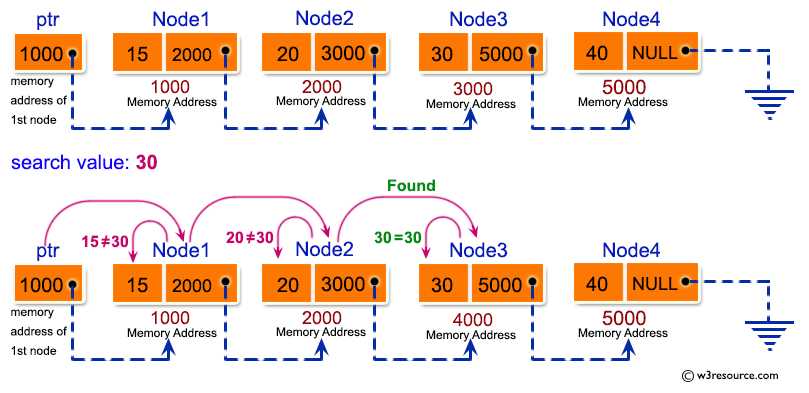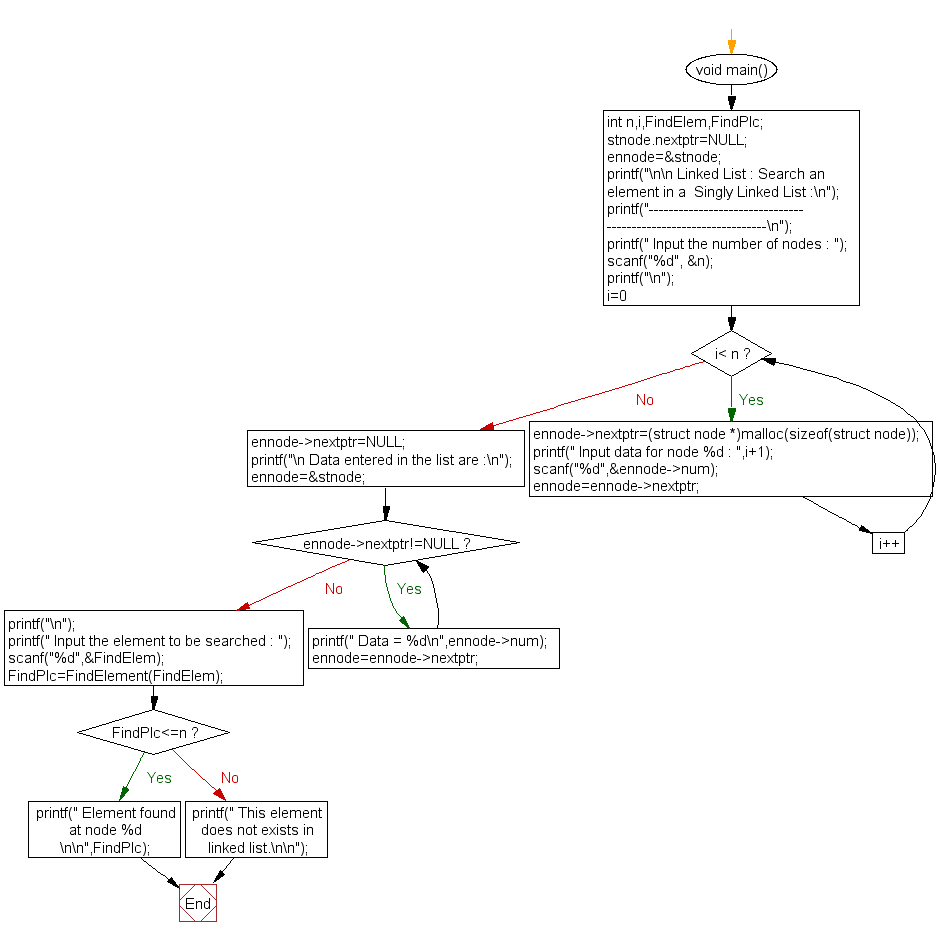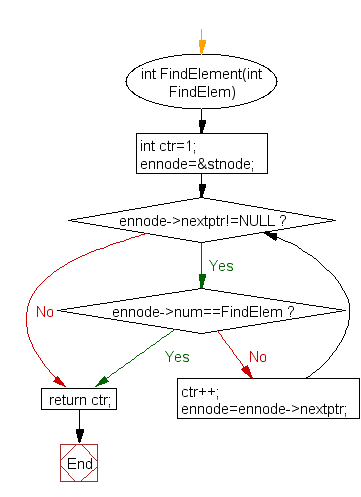﻿ C Program: Search an element in a Singly Linked List - w3resource

# C Exercises: Search an element in a Singly Linked List

## C Singly Linked List : Exercise-10 with Solution

Write a program in C to search for an existing element in a singly linked list.

Pictorial Presentation:Sample Solution:

C Code:

``````#include <stdio.h>
#include <stdlib.h>

struct node
{
int num;
struct node *nextptr;
}

stnode, *ennode;

int FindElement(int);
void main()
{
int n,i,FindElem,FindPlc;
stnode.nextptr=NULL;
ennode=&stnode;
printf("\n\n Linked List : Search an element in a  Singly Linked List :\n");
printf("---------------------------------------------------------------\n");

printf(" Input the number of nodes : ");
scanf("%d", &n);
printf("\n");
for(i=0;i< n;i++)
{
ennode->nextptr=(struct node *)malloc(sizeof(struct node));
printf(" Input data for node %d : ",i+1);
scanf("%d",&ennode->num);
ennode=ennode->nextptr;
}
ennode->nextptr=NULL;
printf("\n Data entered in the list are :\n");

ennode=&stnode;
while(ennode->nextptr!=NULL)
{
printf(" Data = %d\n",ennode->num);
ennode=ennode->nextptr;
}

printf("\n");
printf(" Input the element to be searched : ");
scanf("%d",&FindElem);
FindPlc=FindElement(FindElem);
if(FindPlc<=n)
printf(" Element found at node %d \n\n",FindPlc);
else
printf(" This element does not exists in linked list.\n\n");
}
int FindElement(int FindElem)
{
int ctr=1;
ennode=&stnode;
while(ennode->nextptr!=NULL)
{
if(ennode->num==FindElem)
break;
else
ctr++;
ennode=ennode->nextptr;
}
return ctr;
}
```
```

Sample Output:

``` Linked List : Search an element in a  Singly Linked List :
---------------------------------------------------------------
Input the number of nodes : 3

Input data for node 1 : 2
Input data for node 2 : 5
Input data for node 3 : 8

Data entered in the list are :
Data = 2
Data = 5
Data = 8

Input the element to be searched : 5
Element found at node 2
```

Flowchart:FindElement() :C Programming Code Editor:

What is the difficulty level of this exercise?

Test your Programming skills with w3resource's quiz.

﻿